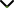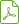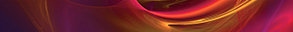# Geometric and Polynomial Approaches of Complex Systems and Control in Mathematics and Applied Sciences

Publishing date
01 Apr 2020
Status
Published
06 Dec 2019

Guest Editors

2Instituto Tecnológico de Tijuana, Baja California, Mexico

# Geometric and Polynomial Approaches of Complex Systems and Control in Mathematics and Applied Sciences

## DescriptionComplex dynamical systems exist in many theoretical and practical domains of science and engineering, including physical processes, man-made systems, networks of leader-follower multiagent systems, and distributed, deterministic, and stochastic control systems.

The matrix approach to state space has long been considered the optimal way of addressing many of the central problems of control systems. In recent decades, novel methods and approaches to the study of these systems, both linear and nonlinear, have been based on a geometrical approach in which the objective is to reveal the properties of the geometric skeleton of the dynamic system. The geometric approach can convert a difficult nonlinear problem into a straightforward linear one.

Geometric control theory and sub-Riemannian geometry are research domains that play an important role in complex dynamical systems, searching controllability, optimality, and stability for linear and nonlinear control systems, applying Lie Theory techniques, Pontryagin Maximum Principle, and other geometric and algebraic techniques to Robotic Control, Motion Planning Problems, sub-Riemannian metric complexity, models of neurobiological visual processing, and digital image reconstruction.

On the other hand, polynomials theory has been a useful tool to explain the classical and complex behavior of dynamical systems, for instance, considering the stability behavior of a dynamical system, a computational search for parameter-dependent transitions can be affected by doing algebraic operations with the coefficients of the characteristic polynomial of the corresponding system. In addition, recently polynomial approaches have been used to study the chaotic behavior of complex systems, particularly to generate scrolls. The polynomial approaches have also been exploited to solve fundamental problems such as controllability, stability, and robustness. Polynomials theory can also be applied in uncertain, nonlinear, time-delay and hybrid systems, and model predictive control.

The goal of this special issue is to develop theoretical and practical methods and tools, useful models, and differential-algebraic and geometric techniques for the analysis of problems in the domain of complex systems. Authors are encouraged to submit papers that discuss new directions in the way of original research in the field of complex systems. Review articles are also encouraged.

Potential topics include but are not limited to the following:

• Optimal control of complex systems
• Polynomial approaches for studying the stability of continuous complex systems
• Control of complex systems and sub-Riemannian geometry
• Polynomial approaches for studying the stability of discrete complex systems
• Control, modeling, and numerical estimation of complex systems
• Polynomial approaches for studying chaotic behavior of complex systems
• Engineering of complex systems and intelligent robotics design
• Sub-Riemannian metric complexity
• Matrix approach for complex systems
• Polynomial approach for control of complex behavior
• Pattern recognition in complex systems
• Analysis and control of complex networksDownload Special IssuePublishing CollaborationJournal metricsAuthor guidelinesEditorial boardDatabases and indexing